# Texas Go Math Grade 8 Lesson 3.1 Answer Key Representing Proportional Relationships

Refer to our Texas Go Math Grade 8 Answer Key Pdf to score good marks in the exams. Test yourself by practicing the problems from Texas Go Math Grade 8 Lesson 3.1 Answer Key Representing Proportional Relationships.

## Texas Go Math Grade 8 Lesson 3.1 Answer Key Representing Proportional Relationships

Explore Activity
Representing Proportional Relationships with Tables
In 1870, the French writer Jules Verne published 20,000 Leagues Under the Sea, one of the most popular science fiction novels ever written. One definition of a league is a unit of measure equaling 3 miles.
A. Complete the table.B. What relationships do you see among the numbers in the table?

C. For each column of the table, find the ratio of the distance in miles to the distance in leagues. Write each ratio in simplest form.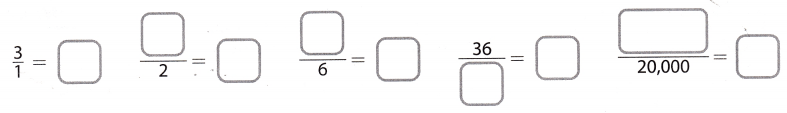D. What do you notice about the ratios? ______________________________________

Reflect

Question 1.
If you know the distance between two points in leagues, how can you find the distance in miles? ______________________________________
Since the definition of a league is that one league is 3 miles to find the distance in miles, multiply the given distance in leagues by 3
We can express this with a mathematical formula:
y = 3x
where y is the distance in miles, and x the distance in leagues.
Multiply by 3

Question 2.
If you know the distance between two points in miles, how can you find the distance in leagues? _________________
Since the definition of a league is that one league is 3 miles to find the distance in miles we have to divide the
distance in miles by 3 (or multiply by $$\frac{1}{3}$$)in order to get the distance in leagues.
We can express this in a mathematical formula:
$$\frac{1}{3}$$y = x
where y is the distance in miles and x is the distance in leagues.

Divide by 3.

Question 3.
Fifteen bicycles are produced each hour at the Speedy Bike Works. Show that the relationship between the number of bikes produced and the number of hours is a proportional relationship. Then write an equation for the relationship. ____________Make a table relating number of bicycle produced to the numbers of hours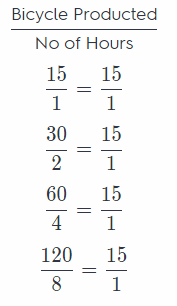For each number of hours, write the relationship of the number of bicycle produced and the number of hours as a ratio in simplest form.
Since the ratios for the two quantities are all equal to $$\frac{15}{1}$$, the relationship is proportional.
y = kx Where an equation Let x represents the number of hours and y represents the bicycle produced
y = 15x

The graph shows the relationship between the amount of time that a backpacker hikes and the distance traveled.

Question 4.
What does the point (5, 6) represent?
The first unit (5) in the point (5, 6) represents the amount of time the backpacker hikes and the second unit (6)
represents the distance traveled.
It means that the backpacker has hiked 6 miles in 5 hours.

The hours (5 h) and distance (6 mi) a backpacker has hiked.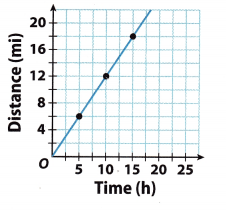Question 5.
What is the equation of the relationship?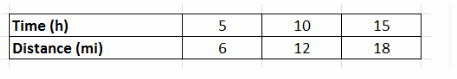Use the points on the graph to make a table.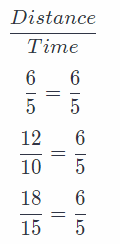Find the constant of proportional
The constant of proportionality $(k)$ is
$$\frac{6}{5}$$

y = kx Write an equation
y = $$\frac{6}{5}$$x Let x represents the number of hours and y represents the distance traveled

Question 1.
Vocabulary A proportional relationship is a relationship between two quantities in which the ratio of one quantity to the other quantityconstant.
A proportional. relationship is a relationship between two quantities in which the ratio of one quantity to the other
quantity is constant

Question 2.
Vocabulary When writing an equation of a proportional relationship in the form y = kx, k represents the ____.
When writing an equation of a proportional relationship in the form y = kx, k represents the constant of proportionality.

Question 3.
Write an equation that describes the proportional relationship between the number of days and the number of weeks in a given length of time. (Explore Activity and Example 1)
a. Complete the table.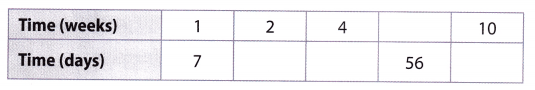Top row: 8, bottom row: 14, 28, 70.

b. Let x represent ______________
Let y represent _____
The equation that describes the relationship is _____
Let x represent the number of weeks in a given length of time.
Let y represent the number of days in a given length of time.
The equation that describes the relationship is y = 7x.

Each table or graph represents a proportional relationship. Write an equation that describes the relationship. (Example 1 and Example 2)

Let x represent weeks, y days, then: y = 7x describes the relationship.

Question 4.
Physical Science The relationship between the numbers of oxygen atoms and hydrogen atoms in water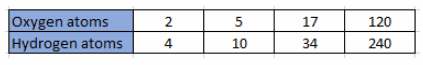To find the constant of proportionality compare the following ratios: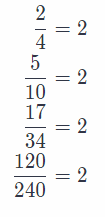The constant of proportionality “k’ is 2.
We can conclude that if y represents the number of hydrogen atoms and æ the number of oxygen atoms in water,
then the following equation describes their relationship:
y = kx
y = 2x

Question 5.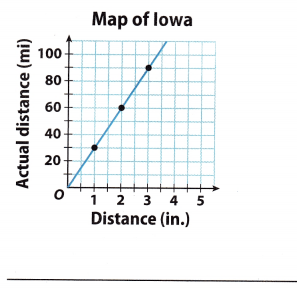Use the points on the graph to make a table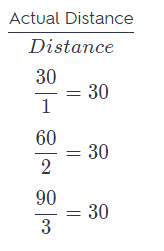Find the constant of proportionality
The constant of proportionality $(k)$ is
30
y = kx Write an equation Let x represents the distance and y represents the actual distance.
y = 30x

Essential Question Check-In

Question 6.
If you know the equation of a proportional relationship, how can you draw the graph of the equation?
If you know the equation of a proportional, relationship, you can substitute few values of x to find y and plot them on a coordinate plane. Connect the points by a straight line.

The table shows the relationship between temperatures measured on the Celsius and Fahrenheit scales.Question 7.
Is the relationship between the temperature scales proportional? Why or why not?
For the relationship between the temperature scales to be proportional, the ratio (the number we get when dividing the corresponding temperature in Celsius by the temperature in Fahrenheit) has to be constant. Then we
call, it a constant of proportionality
$$\frac{0}{32}$$ = 0
$$\frac{10}{50}$$ = 0.2
$$\frac{20}{68}$$ ≈ 0.29
We can see that that is not the case in our example. Therefore, the relationship is not proportional.

No. Because the ration is not the same (no constant of proportionality).

Question 8.
Describe the graph of the Celsius-Fahrenheit relationship.
The graph is a straight line (with a coefficient $$\frac{9}{5}$$) that intercepts the y-axis in (0, 32)

Straight line that intercepts the y-axis in (0, 32).

Question 9.
Analyze Relationships Ralph opened a savings account with a deposit of $100. Every month after that, he deposited$20 more.
a. Why is the relationship described not proportional?
Because the ratio between two consecutive deposits isn’t constant because of the first 100 dollar deposit
The ratio isn’t constant.

b. How could the situation be changed to make the situation proportional?
He coutd be depositing 100 dollars every month also and not just the first.
Constant deposits every month, including the first

Question 10.
Represent Real-World Problems Describe a real-world situation that can be modeled by the equation y = $$\frac{1}{20}$$x. Be sure to describe what each variable represents.
If we need to pay taxes in the amount of $$\frac{1}{20}$$ = 5 percent of our paycheck, the given equation would model the amount of taxes we need to pay depending on how much we earn. y could represent the tax we need to pay and x our paycheck.

y can be taxes, and x our paycheck.

Look for a Pattern The variables x and y are related proportionally.

Question 11.
When x = 8, y = 20. Find y when x = 42. ____________
Since x and y are related proportiotially, the following equation holds:
$$\frac{y}{x}$$ = k (1)
where k is a constant of proportionality.
Now, from
$$\frac{20}{8}$$ = k
it follows that
k = $$\frac{5}{2}$$ = 2.5
Since the variables are related proportionally, the equation (1) has to hold for every x and y in that relation (for k = 2.5).
$$\frac{y}{42}$$ = 2.5
y = 2.5 × 42 Multiply by 12
y = 105 Solve for y

Question 12.
When x = 12, y = 8. Find x when y = 12. ____________
y = kx Direct variation equation relating x and y
k = $$\frac{y}{x}$$ = $$\frac{8}{12}$$ = $$\frac{2}{3}$$ k is the constant of variation
y = 8
x = 12
Equation is
y = $$\frac{2}{3}$$x

12 = $$\frac{2}{3}$$x When y = 12
x = $$\frac{12 * 3}{2}$$ = 18

Question 13.
The graph shows the relationship between the distance that a snail crawls and the time that it crawls.a. Use the points on the graph to make a table.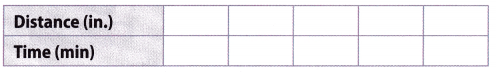Top row: 10, 20, 30, 40, 50; bottom row: 1, 2, 3, 4, 5.

b. Write the equation for the relationship and tell what each variable represents.
First, find the constant of proportionality: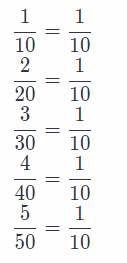The constant of proportionality is k = $$\frac{1}{10}$$
We can conclude that the equation to model the distance that a snail. crawls and the time that it crawls is y = $$\frac{1}{10}$$x
where y represents the time the snail, crawls (in minutes) and x the distance it has crawled (in inches).

y = $$\frac{1}{10}$$x where y is time and x is distance.

c. How long does it take the snail to crawl 85 inches? ________________
In this case, x = 85, therefore:
y = $$\frac{1}{10}$$ × 85 = $$\frac{85}{10}$$ = 8.5
It would take 8 and a half minutes for a snail, to crawl, 85 inches.

Texas Go Math Grade 8 Lesson 3.1 H.O.T. Focus On Higher Order Thinking Answer Key

Question 14.
Communicate Mathematical Ideas Explain why all of the graphs in this lesson show the first quadrant but omit the other three quadrants.
The graphs in this lesson have time, distance and weight as one (or both) of it’s variables. Since these values are
always positive we can only show the first quadrant

Because the graphs have only variables that are always positive.

Question 15.
Analyze Relationships Complete the table.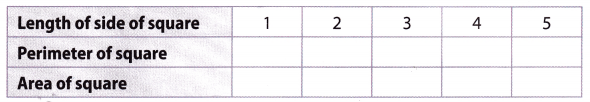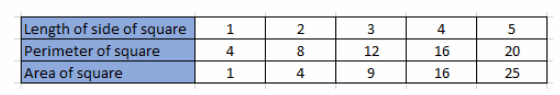a. Are the length of a side of a square and the perimeter of the square related proportionally? Why or why not?
Since the perimeter of a square is 4 times the length of a side of a square the following equation holds:
y = 4x
where y is the perimeter of a square and x is the length of a side of a square. Therefore, they are related proportionally with the constant of proportionality k = 4.
Yes, because their ratio is always the same.

b. Are the length of a side of a square and the area of the square related proportionally? Why or why not?
Lets take a look at some of the ratios:
$$\frac{1}{1}$$ = 1
$$\frac{2}{4}$$ = $$\frac{1}{2}$$
$$\frac{3}{9}$$ = $$\frac{1}{3}$$
Obviously, the ratio isn’t the same (there is no constant of proportionality) and because of that they are not proportional.
No, because there is no constant of proportionality

Question 16.
Make a Conjecture A table shows a proportional relationship where k is the constant of proportionality. The rows are then switched. How does the new constant of proportionality relate to the original one?
$$\frac{y}{x}$$ = k
$$\frac{x}{y}$$ = $$\frac{1}{k}$$